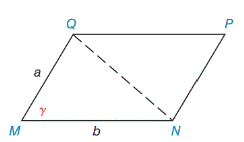Chapter 11.4, Problem 43EElementary Geometry For College St...

7th Edition
Alexander + 2 others
ISBN: 9781337614085

Solutions

Chapter
SectionElementary Geometry For College St...

7th Edition
Alexander + 2 others
ISBN: 9781337614085
Textbook Problem

Find the area of ▱ M N P Q  if  a = 6.3  cm,  b = 8.9  cm, and  γ = 67.5 ∘ . Answer to the nearest tenth of a square centimeter. (See Exercise 41.)Exercises 41 − 44

To determine

To find:

The area of the MNPQ

Explanation

Formula:

The area of the parallelogram is given by,

A=absinγ unit2.

Where, a is the measure of a sides, and b is the base of the triangle,

γ is the angle between the base and the side.

Calculation:

Given,

The area of the MNPQ is given by,

A=absinγ unit2.

Here, a=6.3cm, b=8.9cm, and γ=67.5

A=(6.3)(8.9)sin67

Still sussing out bartleby?

Check out a sample textbook solution.

See a sample solution

The Solution to Your Study Problems

Bartleby provides explanations to thousands of textbook problems written by our experts, many with advanced degrees!

Get Started

f(x)1x(x+2)(x3)

Applied Calculus for the Managerial, Life, and Social Sciences: A Brief Approach

Solve the equations in Exercises 126. (x+1)(x+2)+(x+1)(x+3)=0

Finite Mathematics and Applied Calculus (MindTap Course List)

In problems 37-48, compute and simplify so that only positive exponents remain. 42.

Mathematical Applications for the Management, Life, and Social Sciences

Evaluate the integral. 36. /4/2cot3xdx

Single Variable Calculus: Early Transcendentals

True or False: n=1(1)nn+14 is a convergent series.

Study Guide for Stewart's Single Variable Calculus: Early Transcendentals, 8th